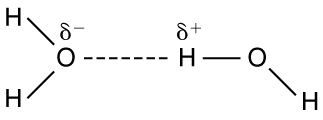# 18.6: Electric Forces in Biology

•• OpenStax
• OpenStax
$$\newcommand{\vecs}{\overset { \rightharpoonup} {\mathbf{#1}} }$$ $$\newcommand{\vecd}{\overset{-\!-\!\rightharpoonup}{\vphantom{a}\smash {#1}}}$$$$\newcommand{\id}{\mathrm{id}}$$ $$\newcommand{\Span}{\mathrm{span}}$$ $$\newcommand{\kernel}{\mathrm{null}\,}$$ $$\newcommand{\range}{\mathrm{range}\,}$$ $$\newcommand{\RealPart}{\mathrm{Re}}$$ $$\newcommand{\ImaginaryPart}{\mathrm{Im}}$$ $$\newcommand{\Argument}{\mathrm{Arg}}$$ $$\newcommand{\norm}{\| #1 \|}$$ $$\newcommand{\inner}{\langle #1, #2 \rangle}$$ $$\newcommand{\Span}{\mathrm{span}}$$ $$\newcommand{\id}{\mathrm{id}}$$ $$\newcommand{\Span}{\mathrm{span}}$$ $$\newcommand{\kernel}{\mathrm{null}\,}$$ $$\newcommand{\range}{\mathrm{range}\,}$$ $$\newcommand{\RealPart}{\mathrm{Re}}$$ $$\newcommand{\ImaginaryPart}{\mathrm{Im}}$$ $$\newcommand{\Argument}{\mathrm{Arg}}$$ $$\newcommand{\norm}{\| #1 \|}$$ $$\newcommand{\inner}{\langle #1, #2 \rangle}$$ $$\newcommand{\Span}{\mathrm{span}}$$$$\newcommand{\AA}{\unicode[.8,0]{x212B}}$$

Learning Objectives

By the end of this section, you will be able to:

• Describe how a water molecule is polar.
• Explain electrostatic screening by a water molecule within a living cell.

Classical electrostatics has an important role to play in modern molecular biology. Large molecules such as proteins, nucleic acids, and so on—so important to life—are usually electrically charged. DNA itself is highly charged; it is the electrostatic force that not only holds the molecule together but gives the molecule structure and strength. Figure $$\PageIndex{1}$$ is a schematic of the DNA double helix.Figure $$\PageIndex{1}$$: DNA is a highly charged molecule. The DNA double helix shows the two coiled strands each containing a row of nitrogenous bases, which “code” the genetic information needed by a living organism. The strands are connected by bonds between pairs of bases. While pairing combinations between certain bases are fixed (C-G and A-T), the sequence of nucleotides in the strand varies. (credit: Jerome Walker)

The four nucleotide bases are given the symbols A (adenine), C (cytosine), G (guanine), and T (thymine). The order of the four bases varies in each strand, but the pairing between bases is always the same. C and G are always paired and A and T are always paired, which helps to preserve the order of bases in cell division (mitosis) so as to pass on the correct genetic information. Since the Coulomb force drops with distance ($$F \propto 1/r^{2}$$), the distances between the base pairs must be small enough that the electrostatic force is sufficient to hold them together.

DNA is a highly charged molecule, with about $$2q_e$$ (fundamental charge) per $$0.3\times 10^{-9}$$m. The distance separating the two strands that make up the DNA structure is about 1 nm, while the distance separating the individual atoms within each base is about 0.3 nm.

One might wonder why electrostatic forces do not play a larger role in biology than they do if we have so many charged molecules. The reason is that the electrostatic force is “diluted” due to screening between molecules. This is due to the presence of other charges in the cell.

## Polarity of Water Molecules

The best example of this charge screening is the water molecule, represented as $$\mathrm{H_{2}O}$$. Water is a strongly polar molecule. Its 10 electrons (8 from the oxygen atom and 2 from the two hydrogen atoms) tend to remain closer to the oxygen nucleus than the hydrogen nuclei. This creates two centers of equal and opposite charges—what is called a dipole, as illustrated in Figure $$\PageIndex{2}$$. The magnitude of the dipole is called the dipole moment.Figure $$\PageIndex{2}$$: This schematic shows water ($$\mathrm{H_{2}O}$$) as a polar molecule. Unequal sharing of electrons between the oxygen (O) and hydrogen (H) atoms leads to a net separation of positive and negative charge—forming a dipole. The symbols $$\delta ^{-}$$ and $$\delta ^{+}$$ indicate that the oxygen side of the $$\mathrm{H_{2}O}$$ molecule tends to be more negative, while the hydrogen ends tend to be more positive. This leads to an attraction of opposite charges between molecules.

These two centers of charge will terminate some of the electric field lines coming from a free charge, as on a DNA molecule. This results in a reduction in the strength of the Coulomb interaction. One might say that screening makes the Coulomb force a short range force rather than long range. Other ions of importance in biology that can reduce or screen Coulomb interactions are $$\mathrm{Na^{+}}$$, and $$\mathrm{K^{+}}$$, and $$\mathrm{Cl^{-}}$$. These ions are located both inside and outside of living cells. The movement of these ions through cell membranes is crucial to the motion of nerve impulses through nerve axons.

Recent studies of electrostatics in biology seem to show that electric fields in cells can be extended over larger distances, in spite of screening, by “microtubules” within the cell. These microtubules are hollow tubes composed of proteins that guide the movement of chromosomes when cells divide, the motion of other organisms within the cell, and provide mechanisms for motion of some cells (as motors).

## Summary

• Many molecules in living organisms, such as DNA, carry a charge.
• An uneven distribution of the positive and negative charges within a polar molecule produces a dipole.
• The effect of a Coulomb field generated by a charged object may be reduced or blocked by other nearby charged objects.
• Biological systems contain water, and because water molecules are polar, they have a strong effect on other molecules in living systems.

## Glossary

dipole
a molecule’s lack of symmetrical charge distribution, causing one side to be more positive and another to be more negative
polar molecule
a molecule with an asymmetrical distribution of positive and negative charge
screening
the dilution or blocking of an electrostatic force on a charged object by the presence of other charges nearby
Coulomb interaction
the interaction between two charged particles generated by the Coulomb forces they exert on one another

This page titled 18.6: Electric Forces in Biology is shared under a CC BY 4.0 license and was authored, remixed, and/or curated by OpenStax via source content that was edited to the style and standards of the LibreTexts platform; a detailed edit history is available upon request.